# A Full Guide to the 30-60-90 Triangle (With Formulas and Examples)

• Author:
• Updated date:

Ray is a licensed engineer in the Philippines. He loves to write about mathematics and civil engineering.

## 30-60-90 Triangle

A 30-60-90 triangle is a unique right triangle. It is an equilateral triangle divided in two from its center down the middle, along with its altitude. A 30-60-90 degree triangle has angle measures of 30°, 60°, and 90°.

A 30-60-90 triangle is a particular right triangle because it has length values consistent and in primary ratio. In any 30-60-90 triangle, the shortest leg is still across the 30-degree angle, the longer leg is the length of the short leg multiplied by the square root of 3, and the hypotenuse's size is always double the length of the shorter leg. In mathematical terms, the previously said properties of a 30-60-90 triangle can be expressed in equations as shown below:

Let x be the side opposite the 30° angle.

• x = side opposite the 30° angle, called the "shorter leg."
• √3 (x) = side opposite the 60° angle or sometimes called the "long leg."
• 2x = side opposite the 90° angle or sometimes called the hypotenuse

### 30-60-90 Triangle Theorem

The 30-60-90 Triangle Theorem states that in a 30-60-90 triangle, the hypotenuse is twice as long as the shorter leg, and the longer leg is the square root of three times as long as the shorter leg.

## 30-60-90 Triangle Theorem Proof

Given triangle ABC with right angle C, angle A = 30°, angle B=60°, BC=a, AC=b, and AB=c. We need to prove that c=2a and b=square root of a.

30-60-90 Triangle Theorem Detailed Proof

StatementsReasons

1. Right triangle ABC with angle A=30°, angle B=60°, and angle C=90°.

1. Given

2. Let Q be the midpoint of side AB.

2. Every segment has precisely one midpoint.

3. Construct side CQ, the median to the hypotenuse side AB.

3. The Line Postulate/Definition of Median of a Triangle

4. CQ = ½ AB

4. The Median Theorem

5. AB = BQ + AQ

5. Definition of Betweenness

6. BQ = AQ

6. Definition of the Median of a Triangle

7. AB = AQ +AQ

7. Law of Substitution

8. AB = 2AQ

9. CQ = ½ (2AQ)

9. Law of Substitution

10. CQ = AQ

10. Multiplicative Inverse

11. CQ = BQ

11. TPE

12. CQ = AQ ; CQ = BQ

12. Definition of Congruent Segments

13. ∠ B = ∠ BCQ

13. The Isosceles Triangle Theorem

14. m∠ B = m∠ BCQ

14. Definition of Congruent Sides

15. m∠ BCQ = 60

15. TPE

16. m∠ B + m∠ BCQ + m∠BQC = 180

16. The sum of the measures of the angles of a triangle is equal to 180.

17. 60 + 60 + m∠ BQC = 180

17. Law of Substitution

18. m∠ BQC = 60

18. APE

19. Triangle BCQ is equiangular and, therefore, equilateral.

19. Definition of an Equiangular Triangle

20. BC = CQ

20. Definition of an Equilateral Triangle

21. BC = ½ AB

21. TPE

To prove that AC = √3BC, we simply apply the Pythagorean Theorem, c2 = a2 + b2.

AB2 = (1/2AB)2 + AC2

AB2 = (AB2)/4 + AC2

(3/4)(AB2) = AC2

(√3/2)AB = AC

√3BC = AC

Scroll to Continue

The theorem previously proven tells us that if we are given a 30-60-90 triangle as in the figure with 2x as the hypotenuse, the legs' lengths are marked.

## 30-60-90 Triangle Formula and Shortcuts

If one side of a 30-60-90 triangle is known, find the other two missing sides by following a pattern formula. Below are three different types and conditions commonly encountered while solving 30-60-90 triangle problems.

• Given the shorter leg, "a."

The longer side's measure is the length of the shorter leg multiplied by √3, and the size of the hypotenuse is double the length of the shorter leg.

• Given the longer leg, "b."

The shorter side's measure is longer leg divided by √3, and the hypotenuse is longer leg multiplied by 2/√3.

• Given the hypotenuse, "c."

The shorter leg's measure is the hypotenuse length divided by two, and the longer leg is the measure of hypotenuse multiplied by √3/2.

## Example 1

### Finding the Measure of the Missing Sides in the 30-60-90 Triangle Given the Hypotenuse

Find the measure of the missing sides given the measurement of the hypotenuse. Given the longest side c = 25 centimeters, find the length of the shorter and longer legs.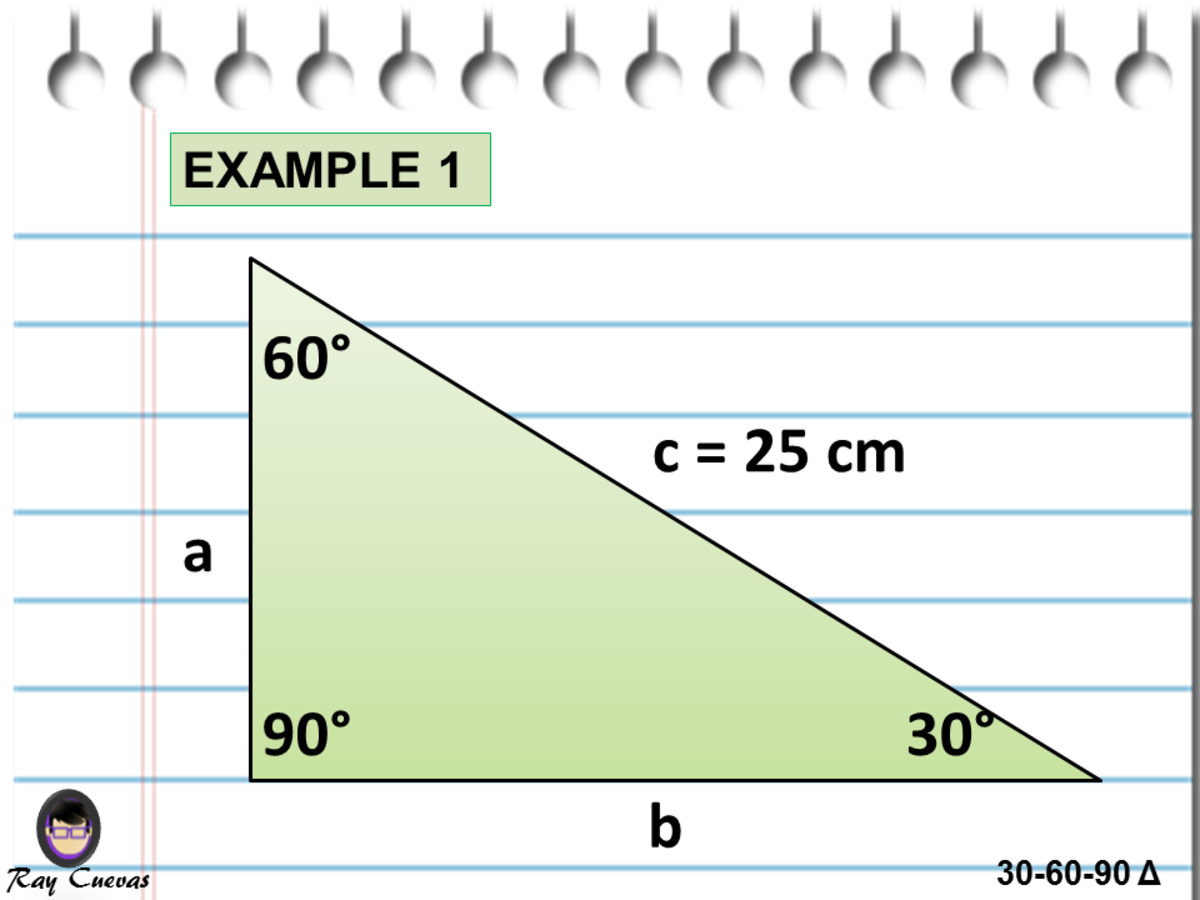Finding the Measure of the Missing Sides in the 30-60-90 Triangle Given the HypotenuseJohn Ray Cuevas

### Solution

Using the shortcut pattern formulas, the formula in solving the short leg given the measure of the hypotenuse is:

a = (1/2) (c)

a = (1/2) (25)

a = 12.5 centimeters

Use the shortcut pattern formulas provided earlier. The formula for solving the long leg is half the hypotenuse multiplied by √3.

b = (1/2) (c) (√3)

b = (1/2) (25) (√3)

b = 21.65 centimeters

The shorter leg is a = 12.5 centimeters, and the longer leg b = 21.65 centimeters.

## Example 2

### Finding the Measure of the Missing Sides in the 30-60-90 Triangle Given the Shorter Leg

Find the measure of the missing sides shown below. Given the length measure of the shorter leg a = 4, find b and c.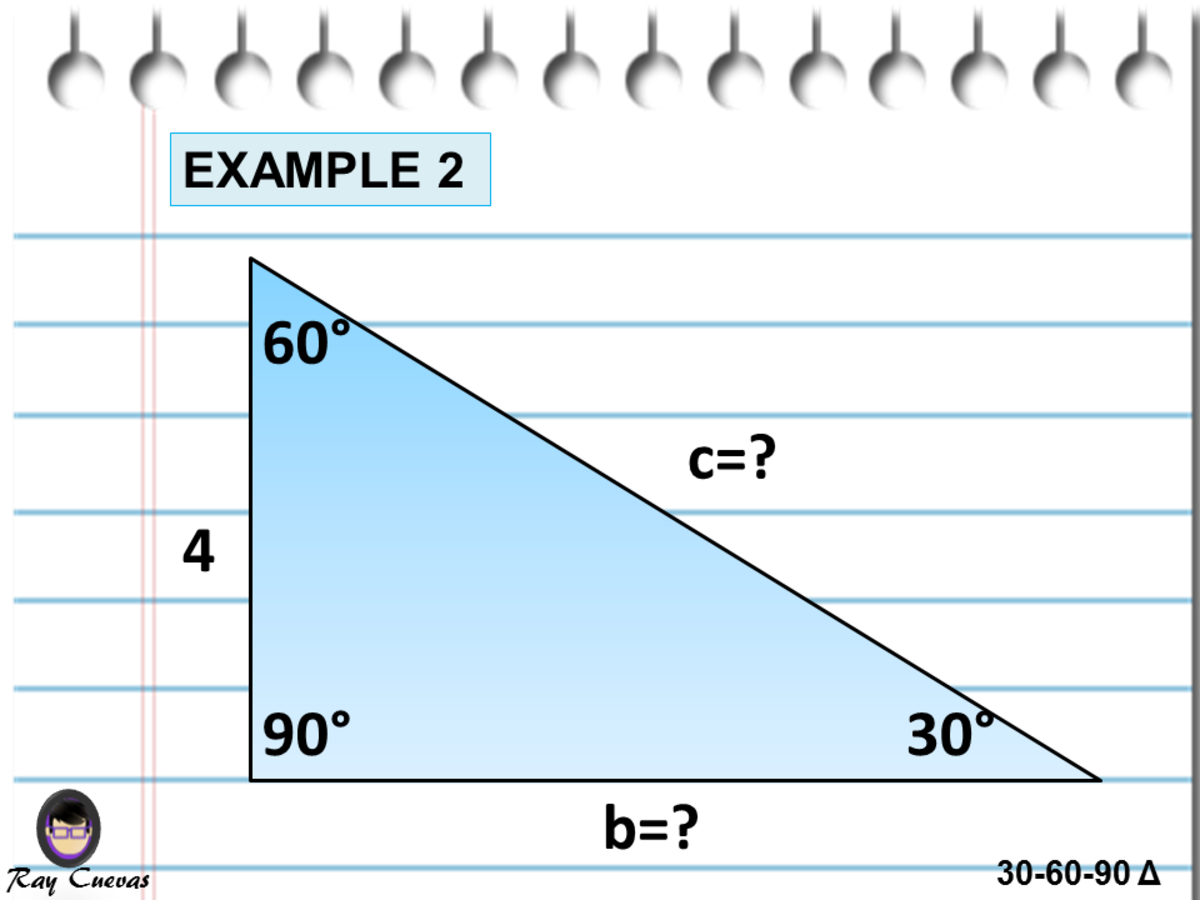Finding the Measure of the Missing Sides in the 30-60-90 Triangle Given the Shorter LegJohn Ray Cuevas

Solution

Let us solve the longest side/hypotenuse c by following the 30-60-90 Triangle Theorem. Recall that the theorem states hypotenuse c is twice as long as the shorter leg. Substitute the value of the shorter leg in the formula.

c = 2(a)

c = 2(4)

c = 8 units

According to the 30-60-90 Triangle Theorem, the longer leg is the square root of three times as long as the shorter leg. Multiply the measure of the shorter leg a = 4 by √3.

b = √3 (a)

b = √3 (4)

b = 4√3 units

The values of the missing sides are b = 4√3 and c = 8.

## Example 3: Finding the Altitude of an Isosceles Right Triangle Using the 30-60-90 Triangle Theorem

Compute the length of the given triangle's altitude below, given the length measure of the hypotenuse c = 35 centimeters.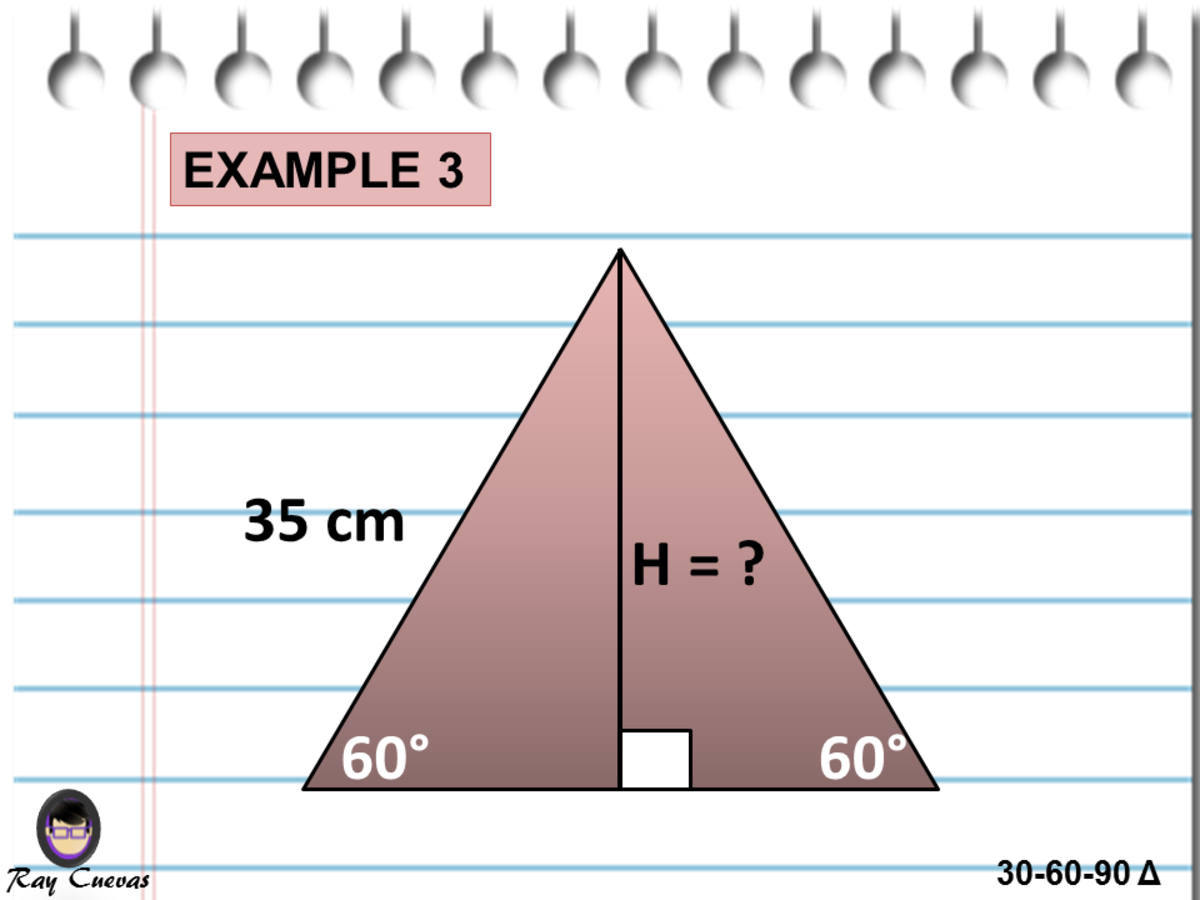Finding the Altitude of an Isosceles Right Triangle Using the 30-60-90 Triangle TheoremJohn Ray Cuevas

Solution

As shown from the picture above, the given side is the hypotenuse, c = 35 centimeters. The altitude of the given triangle is the longer leg. Solve for b by applying the 30-60-90 Triangle Theorem.

H = (1/2) (c) (√3)

H = (1/2) (35) (√3)

H = 30.31 centimeters

The length of the altitude is 30.31 centimeters.

## Example 4: Finding the Altitude of an Isosceles Right Triangle Using the 30-60-90 Triangle Theorem

Compute the length of the given triangle's altitude below given the angle 30° and one side's size, 27√3.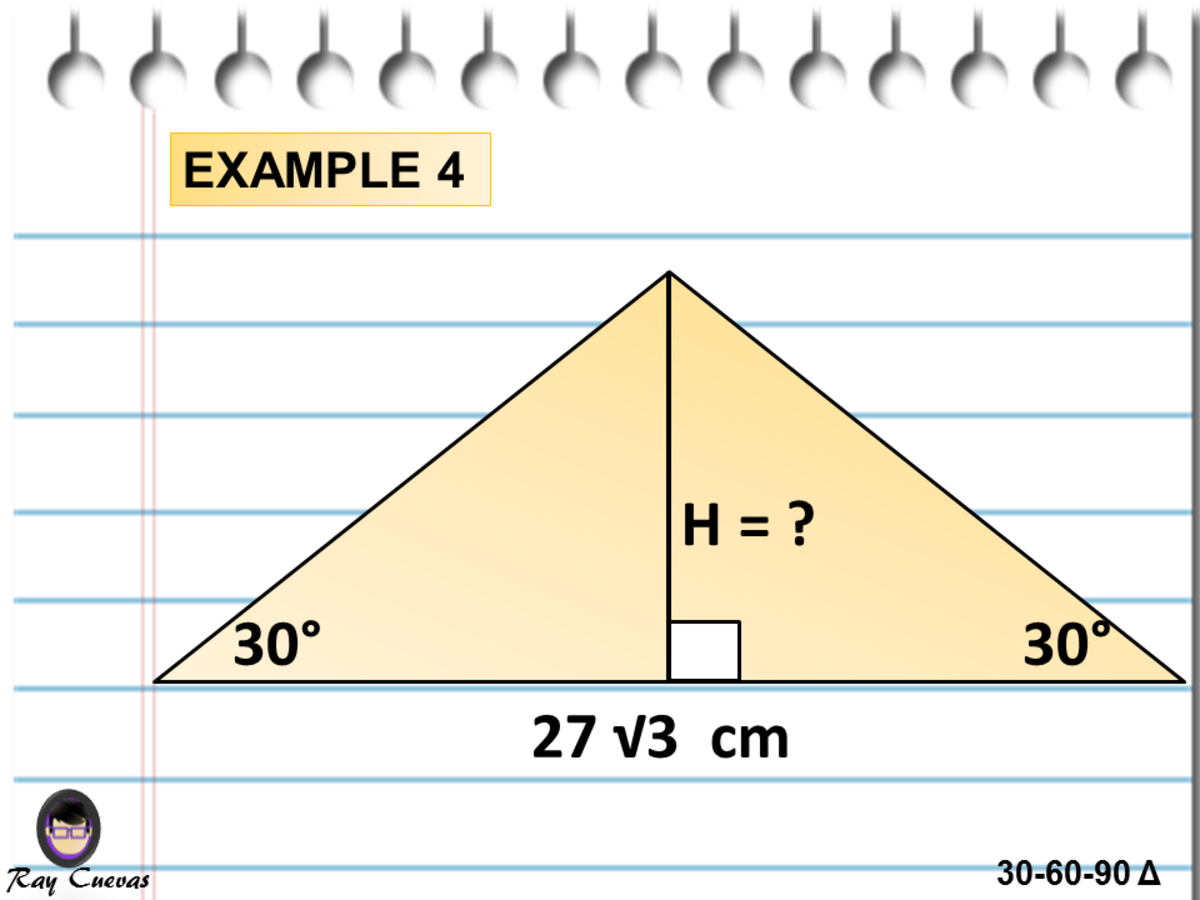Finding the Altitude of an Isosceles Right Triangle Using the 30-60-90 Triangle TheoremJohn Ray Cuevas

Solution

From the two separated right triangles, two pieces of 30-60-90 triangles formed. The given triangle's altitude is the shorter leg since it is the side opposite the 30°. First, solve for the measure of the longer leg b.

b = s/2

b = [(27√3)/2] centimeters

Solve for the altitude or the shorter leg by dividing the longer leg length by √3.

a = [(27√3)/2] / √3

a = 27/2

a = 13.5 centimeters

The altitude of the given triangle is 13.5 centimeters.

## Example 5: Finding the Missing Sides Given One Side of a 30-60-90 Triangle

Use the figure below to compute for the measure of the missing sides of the 30-60-90 triangle.

1. If c = 10, find a and b.
2. If b = 11, find a and c.
3. If a = 6, find b and c.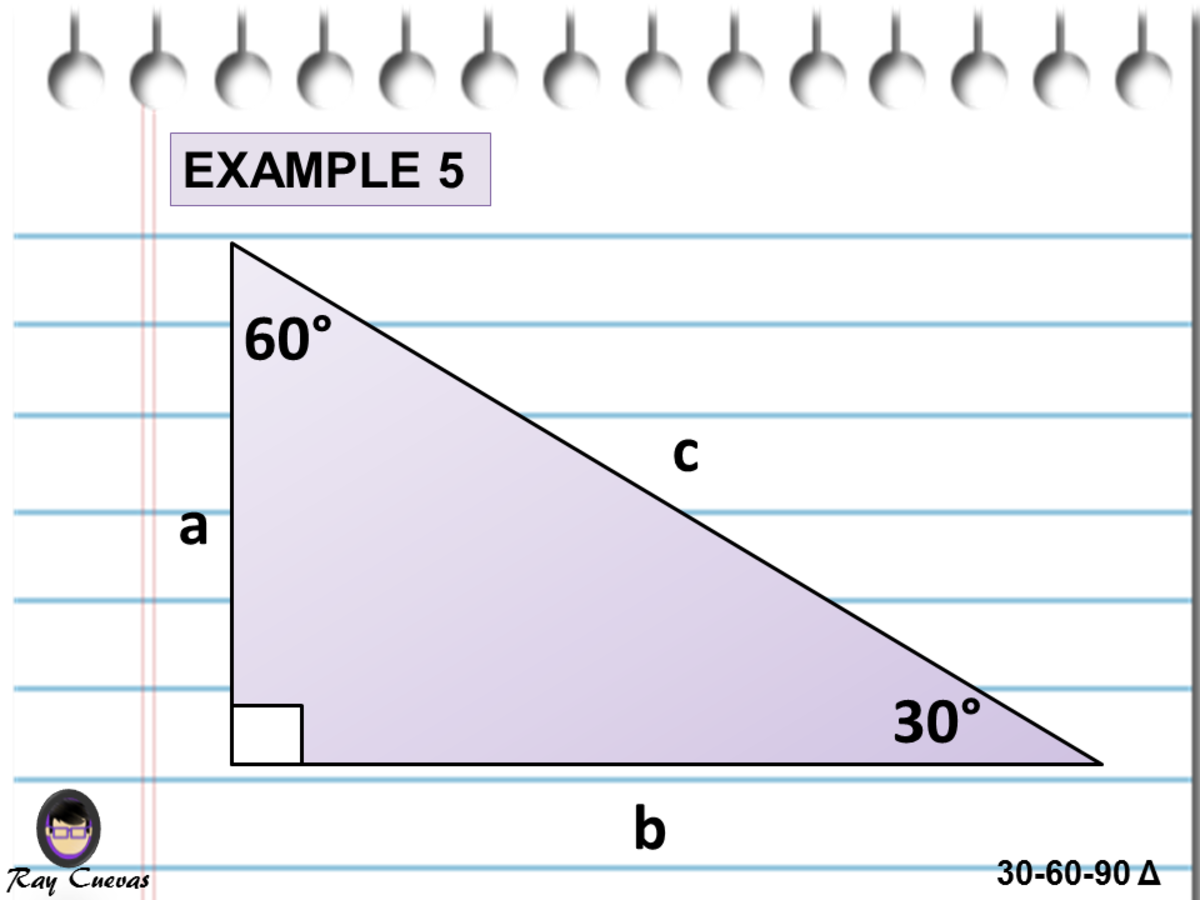Finding the Missing Sides Given One Side of a 30-60-90 TriangleJohn Ray Cuevas

Solution

Note that the given c is the hypotenuse of the triangle. Using the shortcut pattern formulas, solve for a and b.

a = c/2

a = 10/2

a = 5 units

b = (c/2) (√3)

b = (10/2) (√3)

b = 5√3 units

Note that the given b is the longer leg of the 30-60-90 triangle. Using the pattern formulas, solve for a and c. Rationalize the resulting value to get the exact form.

a = b / (√3)

a = 11/√3 units

c = (2/√3) (b)

c = (2/√3) (11)

c = 22/√3

c = (22√3)/3 units

The given value is the shorter leg of the 30-60-90 triangle. Using the 30-60-90 Triangle Theorem, solve for the value of b and c.

b = √3 (a)

b = 6√3 units

c = 2a

c = 2(6)

c = 12 units

1. a = 5 units and b = 5√3 units
2. a = 11√3 units and c = (22√3)/3 units
3. b = 6√3 units and c = 12 units

## Example 6: Finding the Measure of the Missing Sides Given a Complex Triangle

Given ΔABC with angle C a right angle and side CD = 9 is an altitude to the base AB, find AC, BC, AB, AD, and BD using the pattern formulas and 30-60-90 Triangle Theorem.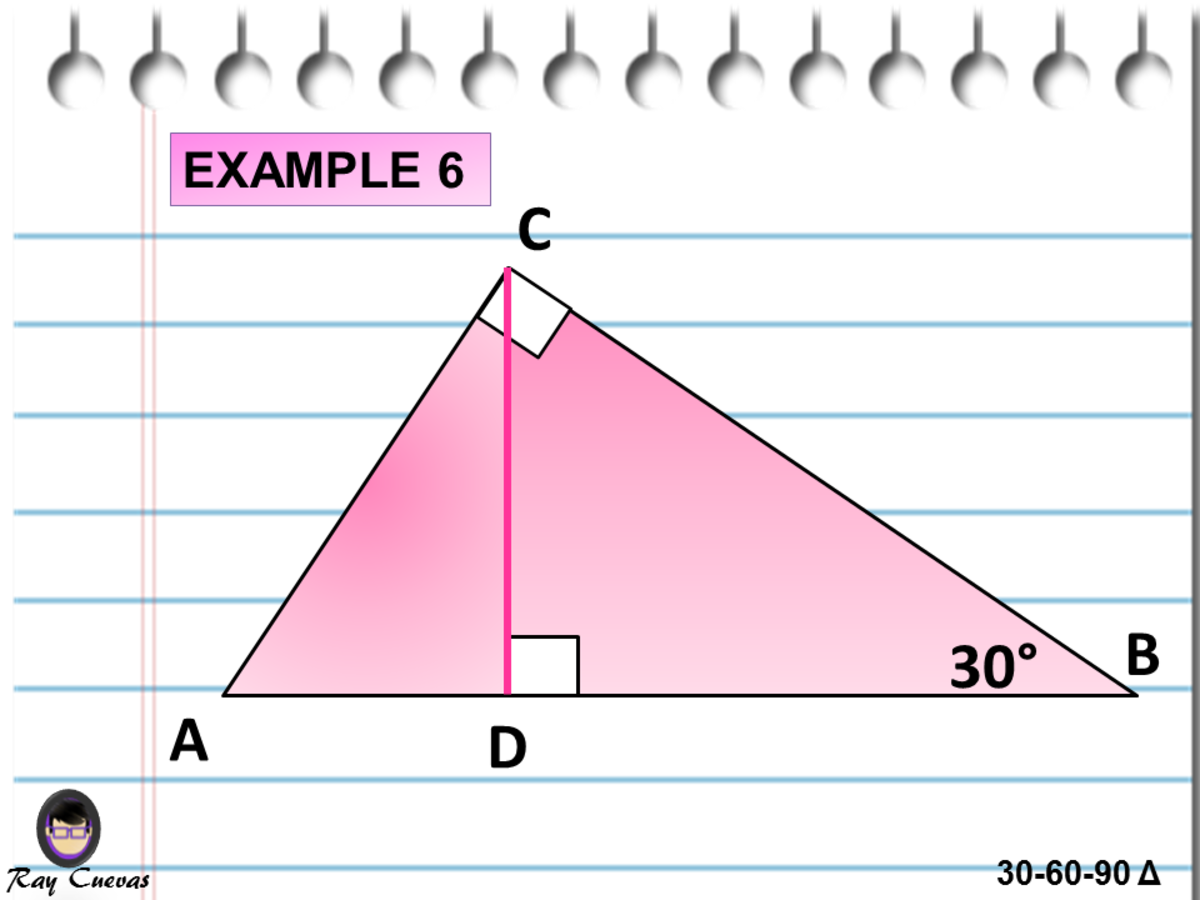Finding the Measure of the Missing Sides Given a Complex TriangleJohn Ray Cuevas

Solution

The two triangles making up the whole triangular figure are 30-60-90 triangles. Given CD = 9, solve AC, BC, AB, AD, and BD using the shortcut patterns and the 30-60-90 Triangle Theorem.

Take note that angle C is a right angle. Given the angle measure of B = 30°, the angle measure of the portion of angle C in ΔBCD is 60°. It makes the remaining angle portion in ΔADC a 30-degree angle.

In ΔADC, the side CD is the longer leg "b." Given CD = b = 9, start with AC, which is the hypotenuse of ΔADC.

AC = 2b/ √3

AC = 2(9)/ √3

AC = 18/√3

AC = 6√3 units

In ΔBCD, the side CD is the shorter leg "a." Solve for BC, the hypotenuse in the ΔBCD.

BC = 2a

BC = 2(9)

BC = 18 units

Solve for AD, which is the shorter leg in the ΔACD.

AD = 9 / √3 units

Solve for BD, which is the longer leg in the ΔBCD.

BD = (√3) a

BD = (√3) (9)

BD = 9√3 units

Add the results in 3 and 4 to get the value of AB.

AB = [9 / √3] + [9√3]

AB = 12√3 units

The final answers are AC = 6√3 units, BC = 18 units, AD = 9 / √3 units, BD = 9√3 units, and AB = 12√3 units.

## Example 7: Trigonometric Application of 30-60-90 Triangle

How long is the ladder, which makes an angle of 30° with the house's side and whose base rests 250 centimeters from the house's toe?

Solution

Use the diagram shown above to solve the 30-60-90 triangle problem. Using the 30-60-90 Triangle Theorem and given b = 250 centimeters, solve for x.

b = x/2

250 = x/2

Using the Multiplication Property of Equality, solve for x.

x = 250 (2)

x = 500 centimeters.

Therefore, the ladder is 500 centimeters long.

## Example 8: Finding the Altitude of an Equilateral Triangle Using the 30-60-90 Triangle Theorem

How long is the altitude of an equilateral triangle whose sides are 9 centimeters each?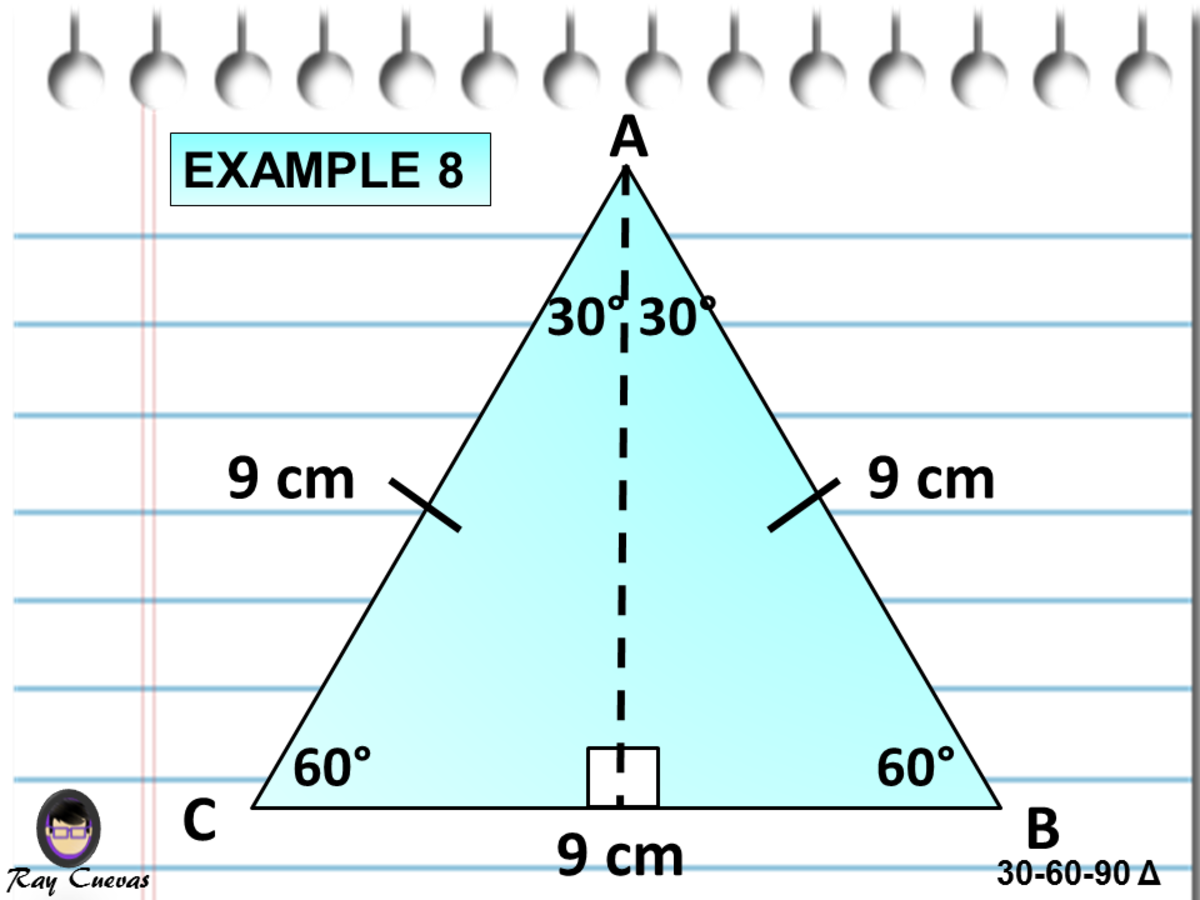Finding the Altitude of an Equilateral Triangle Using the 30-60-90 Triangle TheoremJohn Ray Cuevas

Solution

Construct an altitude from A and name it to side AQ, just like in the figure above. Remember that in an equilateral triangle, a height is also a median and an angle bisector. Therefore, triangle AQC is a 30-60-90 triangle. From this, solve AQ.

AQ = [(9)(√3)] / 2

AQ = 7.794 centimeters

Therefore, the altitude of the triangle is 7.8 centimeters.

## Example 9: Finding the Area of Two 30-60-90 Triangles

Find the area of an equilateral triangle whose sides are "s" centimeters each long.

Solution

Using the formula of area of a triangle bh/2, we have b = "s" centimeters and h = (s/2) (√3). By substitution, the resulting answer is:

A = [(s) (s/2) (√3)] / 2

Simplify the obtained equation above. The final derived equation is the direct formula used when given the side of an equilateral triangle.

A = [s2 (√3)] / [(2)(2)]

A = [s2 (√3)] / 4

The given equilateral triangle area is [s2 (√3)] / 4.

## Example 10: Finding the Length of Sides and Area of an Equilateral Triangle Using the 30-60-90 Triangle Formulas

An equilateral triangle has an altitude of 15 centimeters. How long is each side, and what is its area?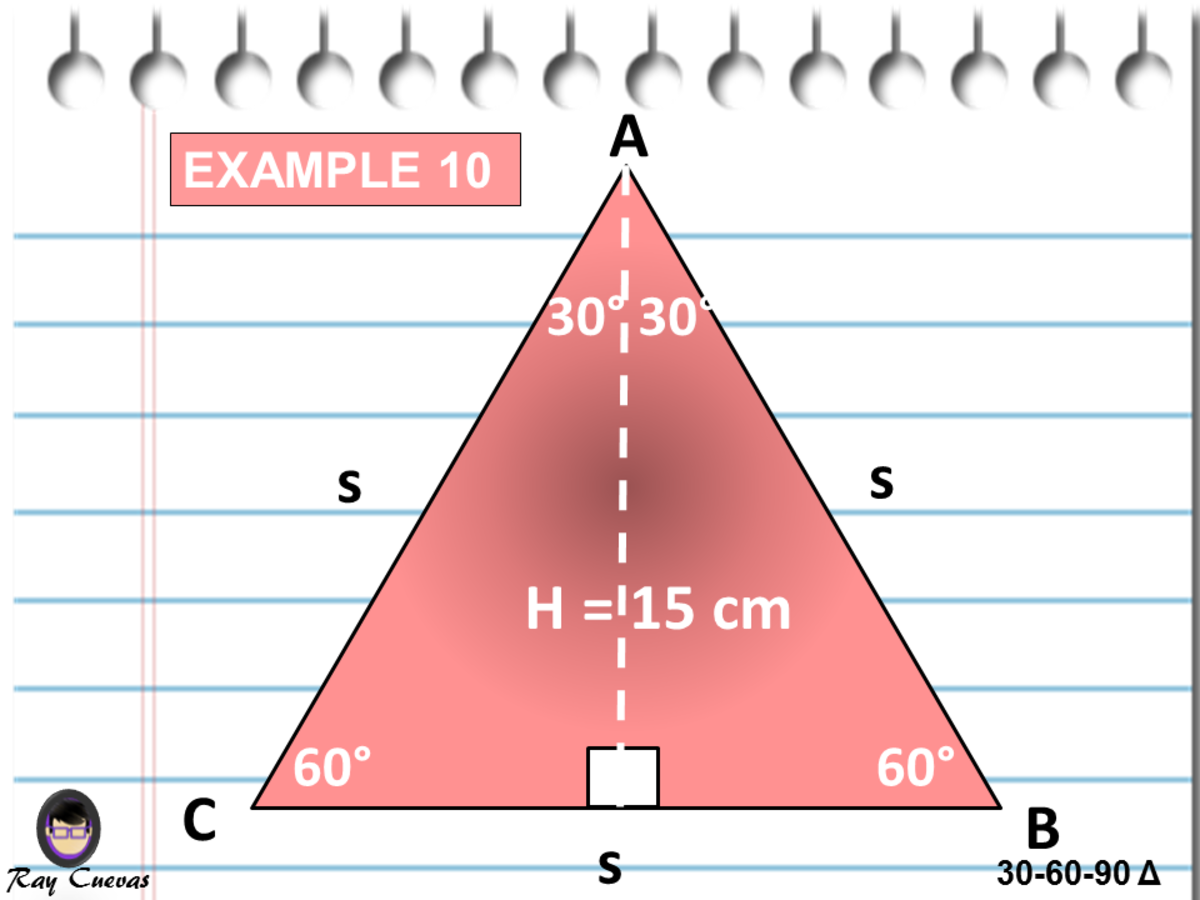Finding the Length of Sides and Area of an Equilateral Triangle Using the 30-60-90 Triangle FormulasJohn Ray Cuevas

Solution

The given altitude is the longer leg of the 30-60-90 triangles. Solve for s.

s = 2b / √3

s = 2(15) / √3

s = 30 / √3

s = 10√3 centimeters

Since the value of s is 10√3 centimeters, substitute the value in the triangle area's formula.

A = (1/2) (s) (b)

A = (1/2) (10√3) (15)

A = 75√3 cm2